Guía de ayuda de MetaTrader 4
Guía de ayuda de MetaTrader 4AnalyticsGraphical ObjectsLine Studies
• Line Studies

# Line Studies

Line studies are lines and various geometrical shapes that can be imposed into a price or indicator chart. They include support/resistance lines, trend lines, as well as Fibonacci's, Gann's, and Andrews' tools, etc.

Line studies are collected in the "Insert" menu and in the "Line Studies" toolbar in terminal. To impose an object, one has to press the corresponding toolbar button (the buttons are given in the leftmost column of the table) or execute a menu command.Description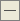Horizontal Line

Horizontal line can be used to mark various levels, particularly, those of support/resistance. One point must be set for this object to be imposed.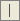Vertical Line

Vertical line can be used to mark various borders in the time axis and to compare signals of indicators to price changes. One point must be set for this object to be imposed.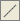Trendline

Trendline helps to explore trends in price changes. Two points must be set through which a trendline will be drawn.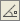Trendline by Angle

Trendline by angle helps to explore trends in price changes. Unlike for a simple trendline, an angle must be set for this line to be drawn. Two points must be set through which a trendline will be drawn.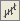Linear Regression Channel

Linear regression is a statistical analysis tool used for forecasting of future values on basis of available data. If the trend is ascending, one can logically suppose that the next bar will be a bit higher than the preceding one. The linear regression method allows to have a statistical demonstration of such logical conclusions. Two points must be set for this tool to be drawn.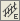Equidistant Channel

Lines of the equidistant channel are always parallel. Two points must be set for this tool to be drawn.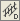Standard Deviation Channel

Standard deviation is the way of volatility measuring based on statistical methods. Standard deviation influences the width of this channel. Two points must be set for this tool to be drawn.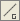Gann Line

Gann Line represents a trendline drawn at an angle of 45 degrees. Two points must be set for this tool to be drawn.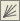Gann Fan

Gann Fan represents a set of trendlines drawn from one point at different angles. Gann considered the trendline of 1x1 (45 degrees) for a very important one. If the price curve is above this line, the market should be considered as bull market, if it is below, it is bear market. Gann supposed the ray of 1x1 to be a powerful supporting line at an ascending trend, and breaking of this line – to be an important turn signal. One point must be set for Gann Fan to be drawn.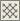Gann Grid

Lines of the Gann Grid are drawn at an angle of 45 degrees. Two points must be set for this tool to be drawn.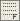Fibonacci Retracement

Leonardo Fibonacci is considered to have discovered a number sequence where each successive number represents a sum of two preceding ones: 1, 1, 2, 3, 5, 8, 13, 21, 34, 55, 89, 144, etc. Each number is approximately 1.618 times more than the preceding one, and each number makes approximately 0.618 of the successive one. The tool can be drawn on two points that determine the trendline. At that, horizontal lines that meet the trendline at Fibonacci levels (retracement) as 0.0%, 23.6%, 38.2%, 50%, 61.8%, 100%, 161.8%, 261.8%, and 423.6% are drawn automatically.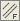Fibonacci Channel

To draw this tool, a channel is used the width of which is taken as one. Then, at the distances defined by the Fibonacci sequence, parallels are drawn starting with the distance of 0.618 of the channel width, then 1.000, 1.618, 2.618, 4.236, etc. Two points and the basic channel width must be set for this tool to be drawn.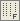Fibonacci Time Zones

Fibonacci Time Zones represent a row of vertical lines placed at Fibonacci intervals from each other: 1, 2, 3, 5, 8, 13, 21, 34, etc. It is considered that significant price changes should be expected near these lines. The tool can be drawn on two points that define the unit interval.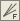Fibonacci Fan

Fibonacci Fan is drawn on two points that define the trendline. Then an "invisible" vertical line is drawn through the second point. Then three trendlines are drawn from the first point, these trendlines meeting the invisible vertical line at Fibonacci levels of 38.2%, 50%, and 61.8%. It is considered that significant price changes should be expected near these lines.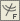Fibonacci Arcs

The tool named Fibonacci Arcs is drawn on two points that define the trendline. Then three arcs having the centers in the second point are drawn, these arcs meeting the trendline at Fibonacci levels of 38.2%, 50%, and 61.8%. It is considered that significant price changes should be expected near these arcs.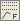Fibonacci Expansion

Fibonacci Expansion is drawn on three points that circumscribe two waves. Then three lines meeting the third, "presumptive", wave at Fibonacci levels of 61.8%, 100%, and 161.8%, are drawn. It is considered that significant price changes should be expected near these lines.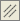Andrews' Pitchfork

This tool is drawn on three points and represents the parallel trendlines. The first trendline starts at the selected leftmost point (it is an important peak or trough) and is drawn precisely between two rightmost points. This line is the pitchfork "helve". Then, the second and the third trendlines outgoing from the above-mentioned rightmost points (significant peak and trough) are drawn in parallel to the first trendline. These lines are the pitchfork "teeth". Andrews' Pitchfork is interpreted as support/resistance lines are normally interpreted.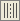Cycle Lines

This tool represents a row of vertical lines placed at equal intervals. Normally, a unit interval corresponds with one cycle. At that, completed lines are considered to describe future cycles. The tool is drawn on two points that define the unit interval.

 The trend lines can be moved in groups within a chart if their node points are located in immediate proximity to each other (within the range of two pixels).